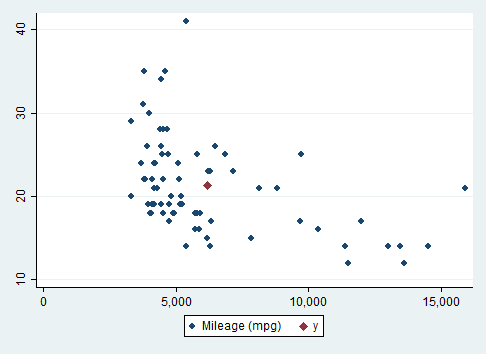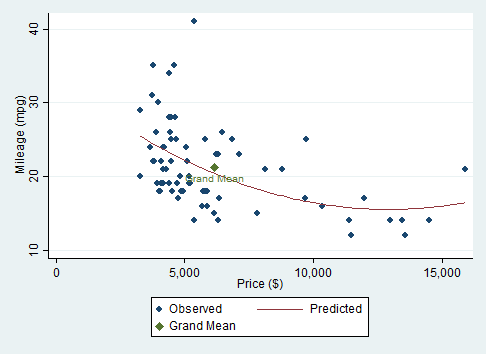Stata notes

There are a couple of approaches one could take to add a single point to a scatterplot. One is to overlay the scatterplot with the plot produced by scatteri, an immediate scatterplot.

In this example, we will plot the overall mean in both the $$x$$ and the $$y$$ variables. A linear regression of $$y$$ on $$x$$ always passes through this point. A regression with higher-order terms seldom passes through this point!

## Identifying the mean

First we identify the mean values of $$y$$ and $$x$$ and save them as local macro variables.

  sysuse auto
summarize price, meanonly
local X = r(mean)
summarize mpg, meanonly
local Y = r(mean)

## Overlay scatter and scatteri

Then we overlay the scatterplot and the immediate scatterplot of the single point.

  twoway (scatter mpg price) (scatteri Y' X', msymbol(D))

The msymbol(D) gives us a large, diamond-shaped point marker.## Label the point, add a regression line

  twoway (scatter mpg price)(lfit mpg price) ///
(scatteri Y' X' (6) "Grand Mean", msymbol(D))

The "(6)" is a clock position for the point label.twoway (scatter mpg price)(qfit mpg price) ///
(scatteri Y' X' (6) "Grand Mean", msymbol(D)), ///
legend(order(1 "Observed" 2 "Predicted" 3 "Grand Mean"))